# Center of mass Wind energy is gaining increased attention, so there is in turn an increased...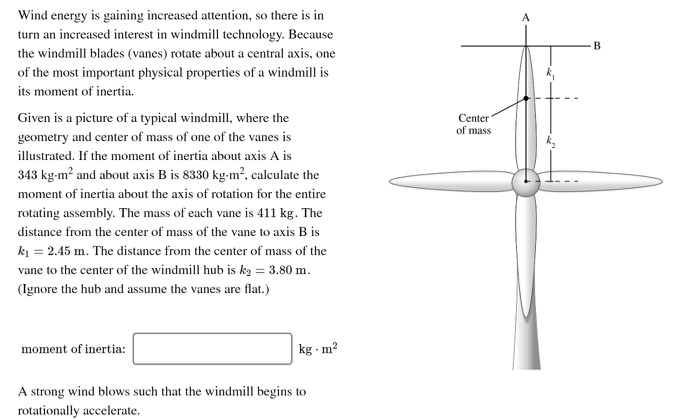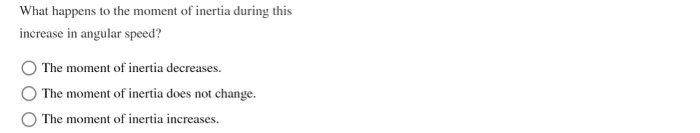Center of mass Wind energy is gaining increased attention, so there is in turn an increased interest in windmill technology. Because the windmill blades (vanes) rotate about a central axis, one of the most important physical properties of a windmill is its moment of inertia. Given is a picture of a typical windmill, where the geometry and center of mass of one of the vanes is illustrated. If the moment of inertia about axis A is 343 kg.mand about axis B is 8330 kg.m², calculate the moment of inertia about the axis of rotation for the entire rotating assembly. The mass of each vane is 411 kg. The distance from the center of mass of the vane to axis B is ki = 2.45 m. The distance from the center of mass of the vane to the center of the windmill hub is kg = 3.80 m. (Ignore the hub and assume the vanes are flat.) moment of inertia: kg.m A strong wind blows such that the windmill begins to rotationally accelerate.
What happens to the moment of inertia during this increase in angular speed? The moment of inertia decreases. The moment of inertia does not change. The moment of inertia increases.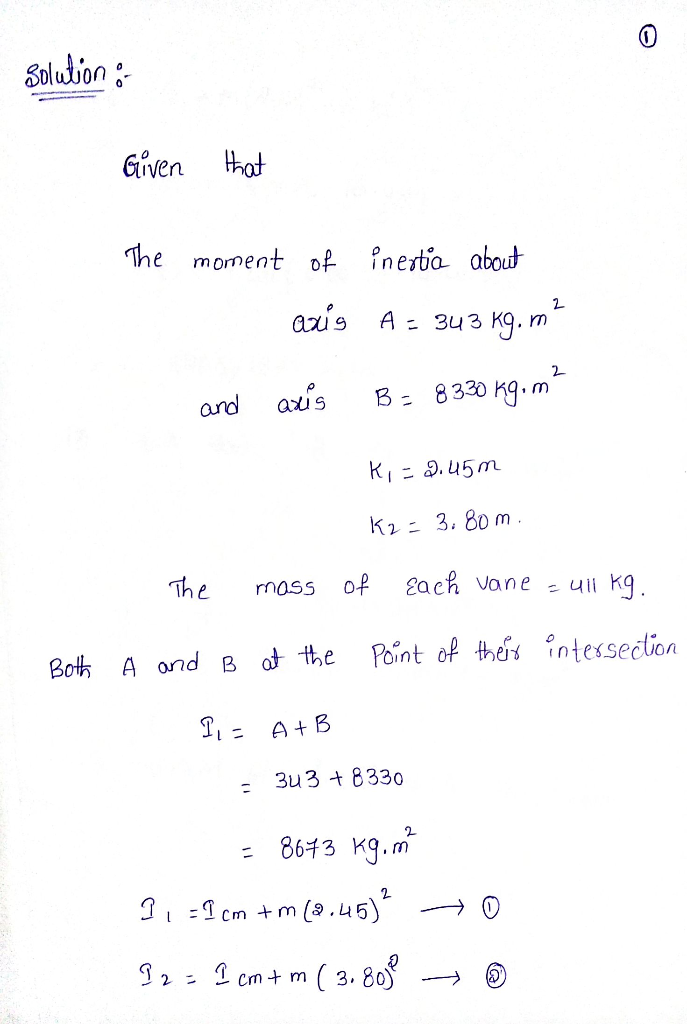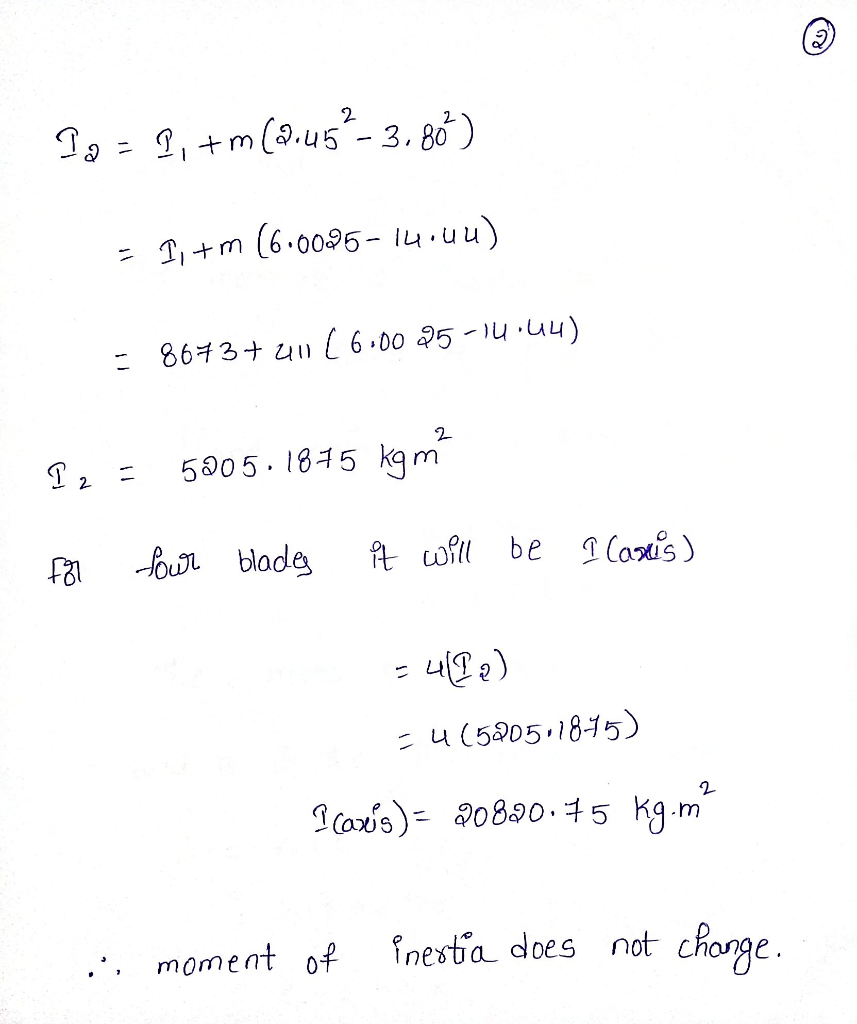please comment if you have any doubt

keep HomeworkLibing ........keep smiling

#### Earn Coin

Coins can be redeemed for fabulous gifts.

Similar Homework Help Questions
• ### Wind energy is gaining increased attention, so there is in turn an increased interest in windmill...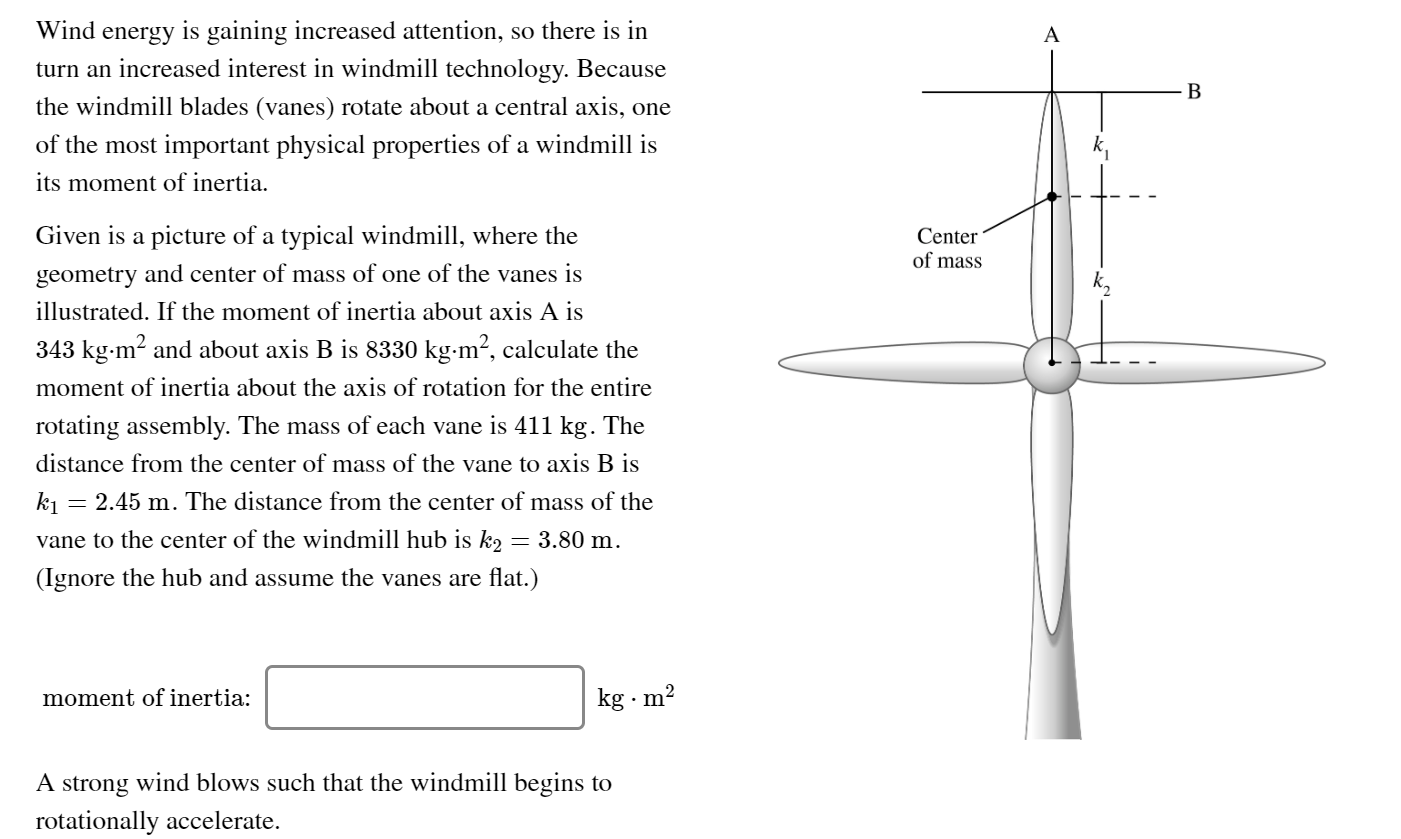Wind energy is gaining increased attention, so there is in turn an increased interest in windmill technology. Because the windmill blades (vanes) rotate about a central axis, one of the most important physical properties of a windmill is its moment of inertia. Center of mass Given is a picture of a typical windmill, where the geometry and center of mass of one of the vanes is illustrated. If the moment of inertia about axis A is 343 kg.m2 and about...

• ### Wind energy is gaining increased attention, so there is in turn an increased interest in windmill...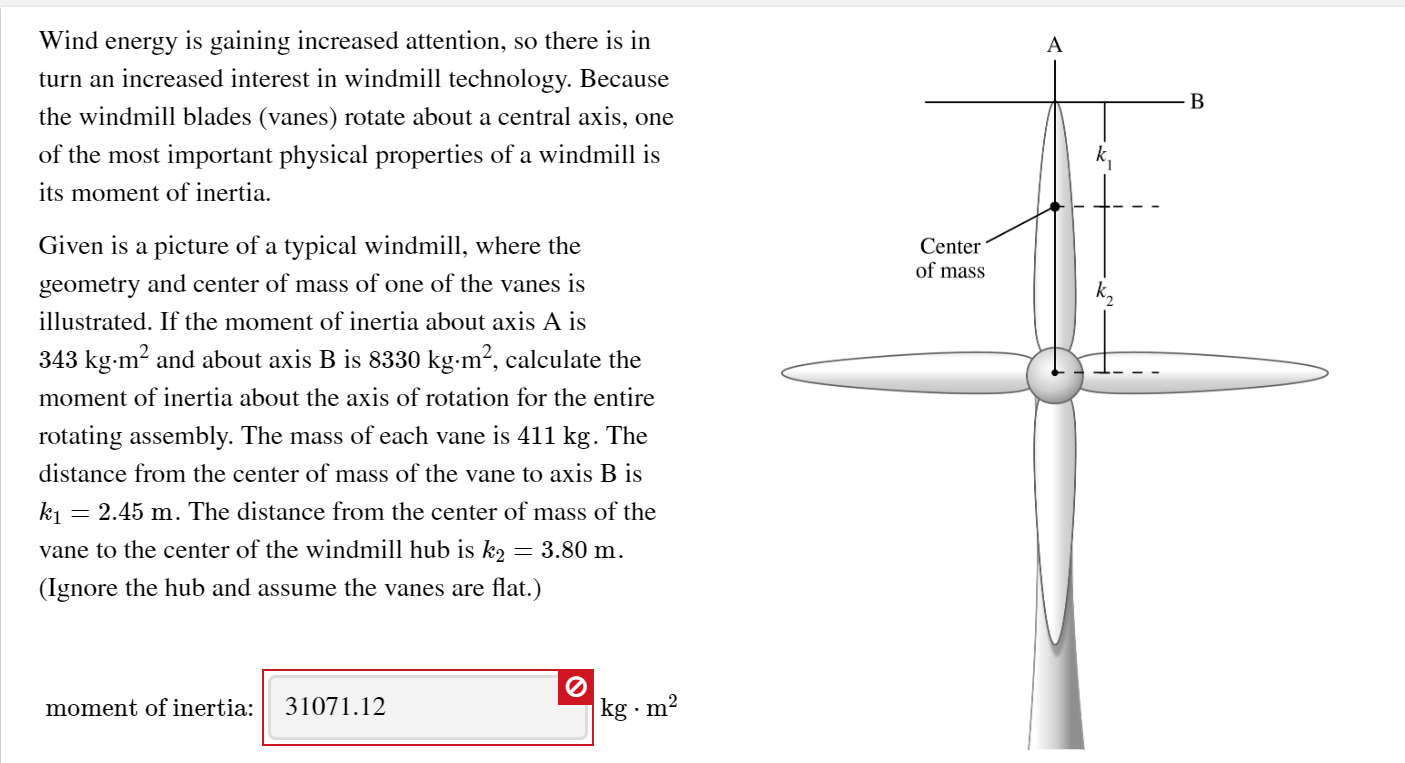Wind energy is gaining increased attention, so there is in turn an increased interest in windmill technology. Because the windmill blades (vanes) rotate about a central axis, one of the most important physical properties of a windmill is its moment of inertia. Center of mass - - - - Given is a picture of a typical windmill, where the geometry and center of mass of one of the vanes is illustrated. If the moment of inertia about axis A is...

• ### A long beam with a length L -0.73 m and mass M - 3.4 kg has...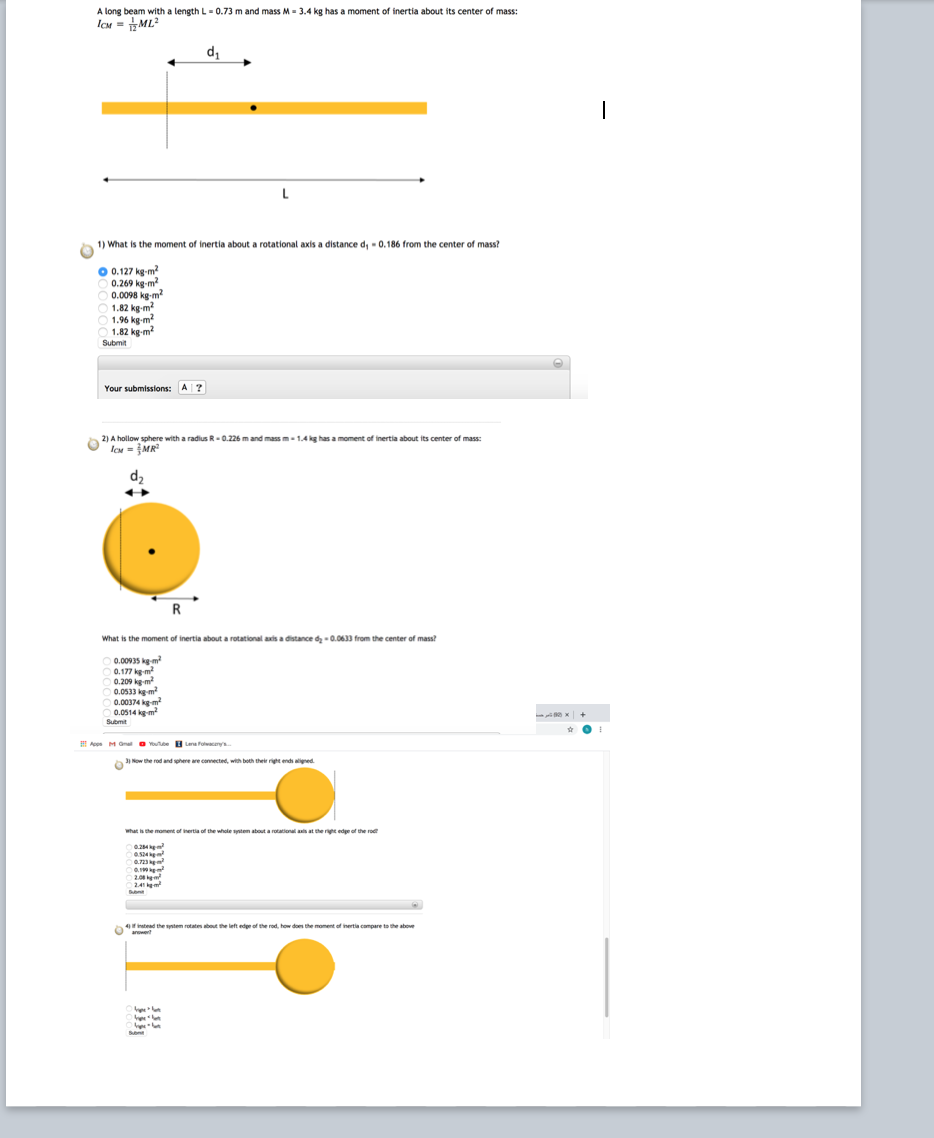A long beam with a length L -0.73 m and mass M - 3.4 kg has a moment of inertia about its center of mass: ICH = 1 ML 1) What is the moment of inertia about a rotational axis a distance d, -0.186 from the center of mass? O 0.127 kg.m 0.269 kg-m 0.0098 kg-m? 1.82 kg-m? 1.96 kg-m? 1.82 kg-m? Submit Your submissions: A ? - 1.4 kg has a moment of inertia about its center of mass:...

• ### help 18. Assuming the lower arm has a mass of 2.8 kg and its center of...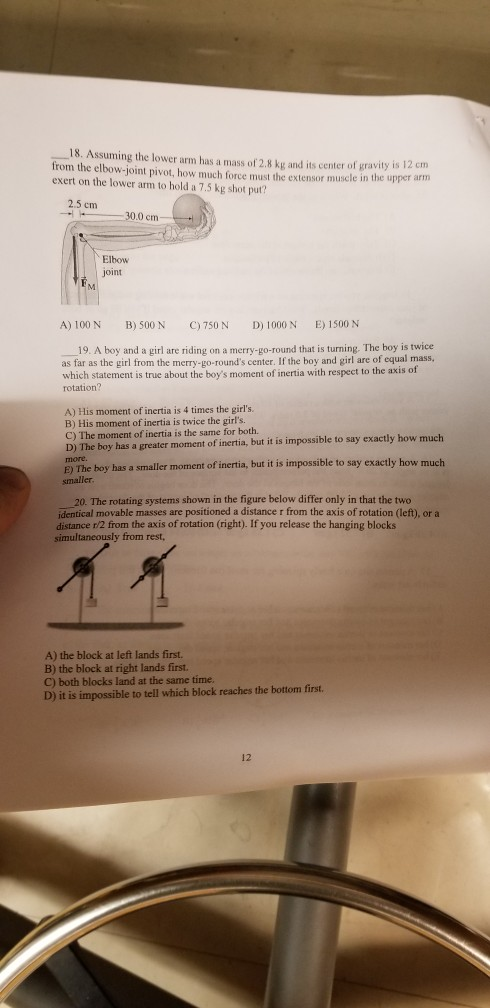help 18. Assuming the lower arm has a mass of 2.8 kg and its center of gravity is 12 cm from the elbow-joint pivot, how much force must the extensor muscle in the upper arm exert on the lower arm to hold a 7.5 kg shot put? 2.5 cm 0 cm- Elbow joint A) 100 N B)500 N C)750 N D) 1000 N E)1500 N 19. A boy and a girl are riding on a merry-go-round that is turning. The...

• ### 18. Assuming the lower arm has a mass of 2.8 kg and its center of gravity...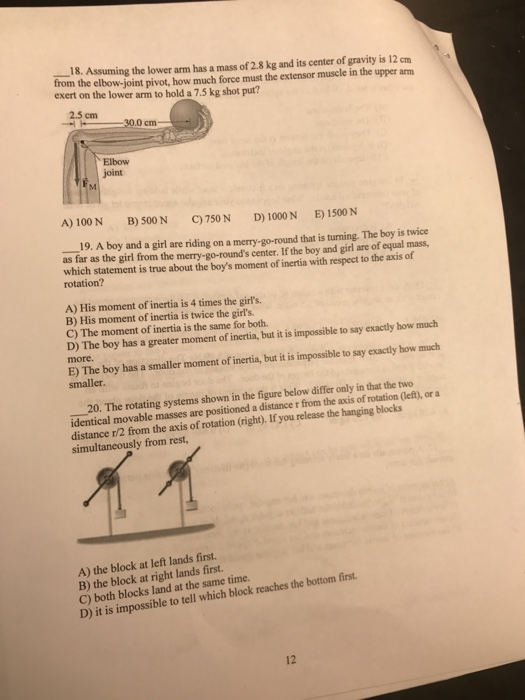18. Assuming the lower arm has a mass of 2.8 kg and its center of gravity is 12 cm from the elbow-joint pivot, how much force must the extensor muscle in the upper arm exert on the lower arm to hold a 7.5 kg shot put? 2.5 cm 30.0 cm- Elbow joint A) 100 N B) 500N C)750N D) 1000 N E) 1500 N 19. A boy and a girl are riding on a merry-go-round that is turning. The boy...

• ### Part A Each blade on a wind turbine is engineered to produce an effective force through its center of mass that is perpendicular to its long axis

Part AEach blade on a wind turbine is engineered to produce an effective force through its center of mass that is perpendicular to its long axis. (Part A figure) The magnitude of the force produced by each blade in a wind of speed v is F=(40.0~{rm kg/s})v. If the center of mass of each blade is r_1 = 11.0 m, what is U_1, the total work done by the wind turbine's blades, in a wind of speed v_1 = 3.90...

• ### Find the moment of inertia of this object about an axis through its center of mass.A spherically symmetric object, with radius R = 0.700 m and mass M = 2.20 kg, rolls without slippingacross a horizontal floor, with velocity V = 2.600 m/s. It then rolls up an incline with an angle of inclinationθ = 40.0 deg and comes to rest a distance d = 0.992 m up the incline, before reversing direction and rolling back down.a) Find the moment of inertia of this object about an axis through its center of mass

• ### PERIOD OF THE LEG The period of the leg can be approximated by treating the leg as a physical pendulum, with a period of 2pi*sqrt(I/mgh), where I is the moment of inertia, m is the mass, and h is the distance from the pivot point to the center of mass

PERIOD OF THE LEGThe period of the leg can be approximated by treating the leg as a physical pendulum, with a period of 2pi*sqrt(I/mgh), where I is the moment of inertia, m is the mass, and h is the distance from the pivot point to the center of mass. The leg can be considered to be a right cylinder of constant density. For a man, the leg constitutes 16% of his total mass and 48% of his total height.Find the...

• ### The rotor (flywheel) of a toy gyroscope has a mass of 0.165 kg. Its moment of...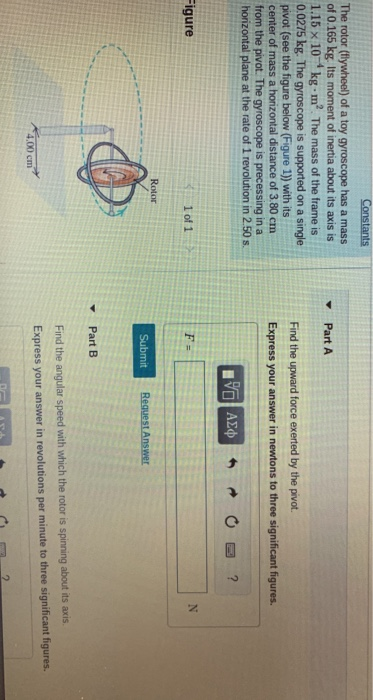The rotor (flywheel) of a toy gyroscope has a mass of 0.165 kg. Its moment of inertia about its axis is 1.15 × 10-4 kg-m2 The mass of the frame is 0.0275 kg. The gyroscope is supported on a single pivot (see the figure below (Figure 1) with its center of mass a horizontal distance of 3.80 cm from the pivot. The gyroscope is precessing in a horizontal plane at the rate of 1 revolution in 2.50 s Part A...

• ### Q17. A metallic sphere of mass 1kg and radius 5 cm is welded to the end...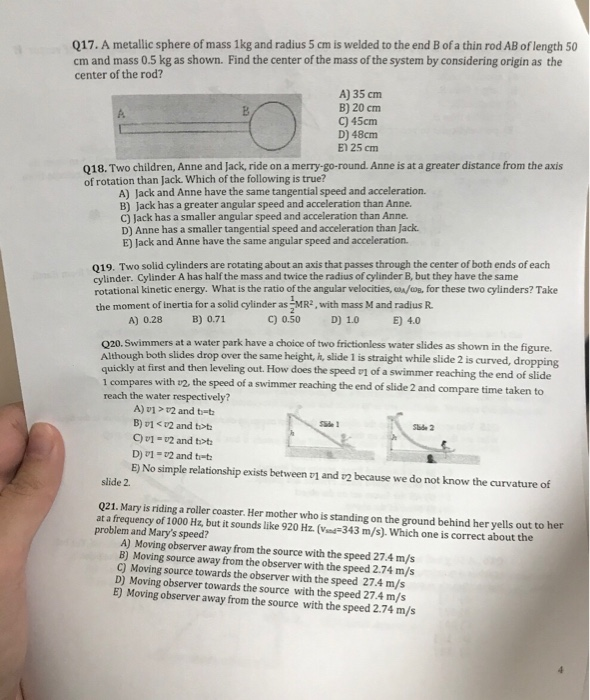Q17. A metallic sphere of mass 1kg and radius 5 cm is welded to the end B of a thin rod AB of length 50 cm and mass o.5 kg as shown. Find the center of the mass of the system by considering origin as the center of the rod? A) 35 cm B) 20 cm C) 45cm D) 48cm E1 25 cm A. Q18. Two children, Anne and Jack, ride on a merry-go-round. Anne is at a greater distance...